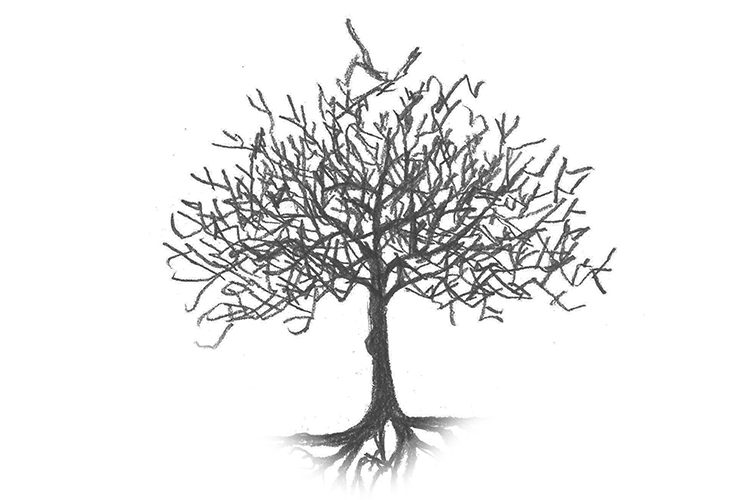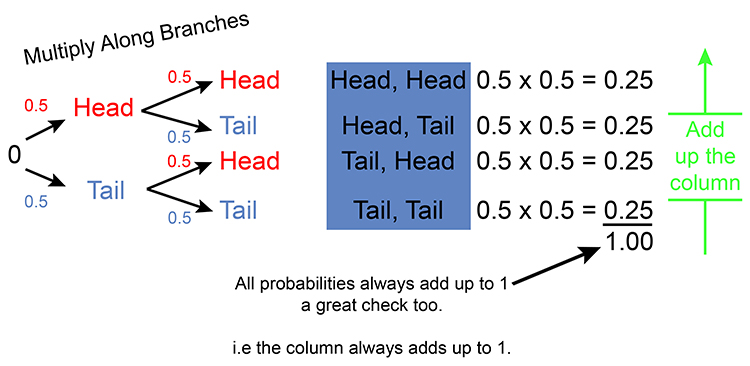# Probability tree

## A tree shaped diagram showing all possible outcomes (looks like a tree with branches)

In order to really achieve an understanding of a question on probability a “Probability Tree” will help enormously.

If faced with a probability question
ALWAYS draw a probability tree

A tree of possible outcomes.A tree with branches, relates to a real probability tree which looks like: